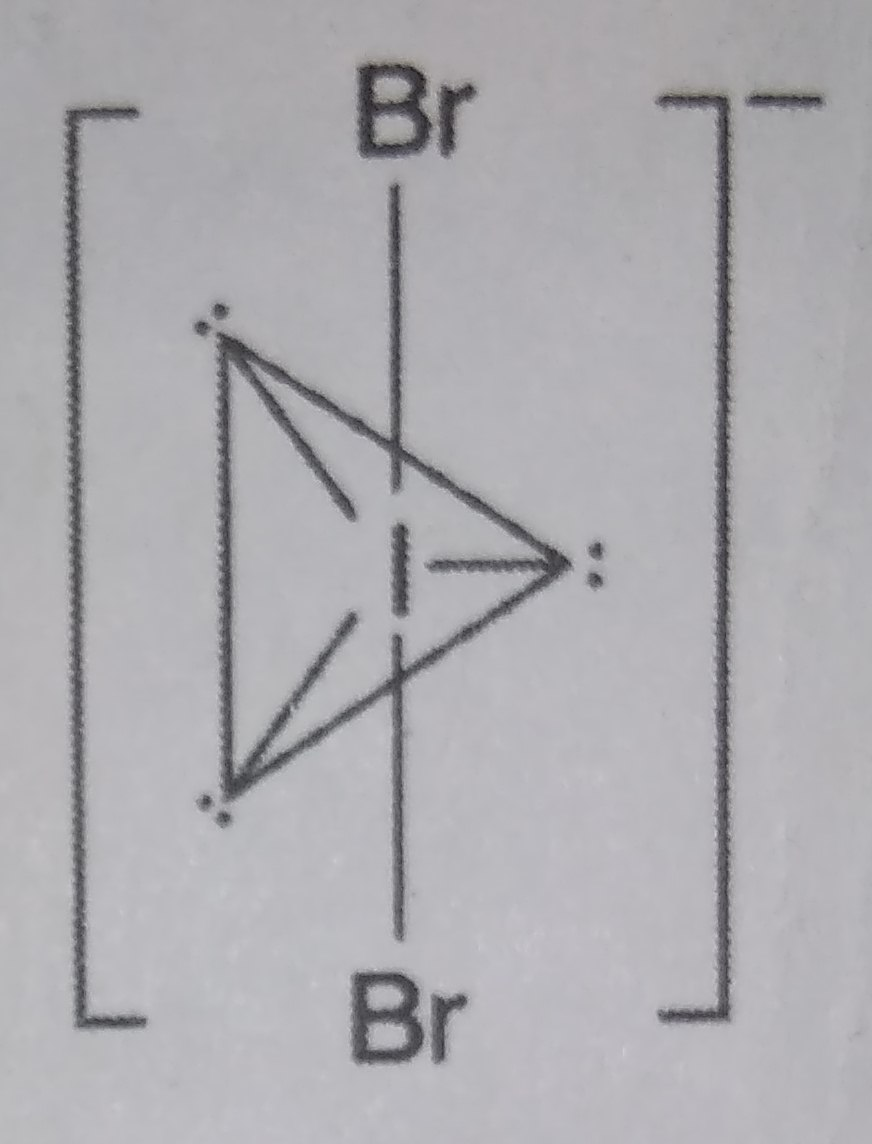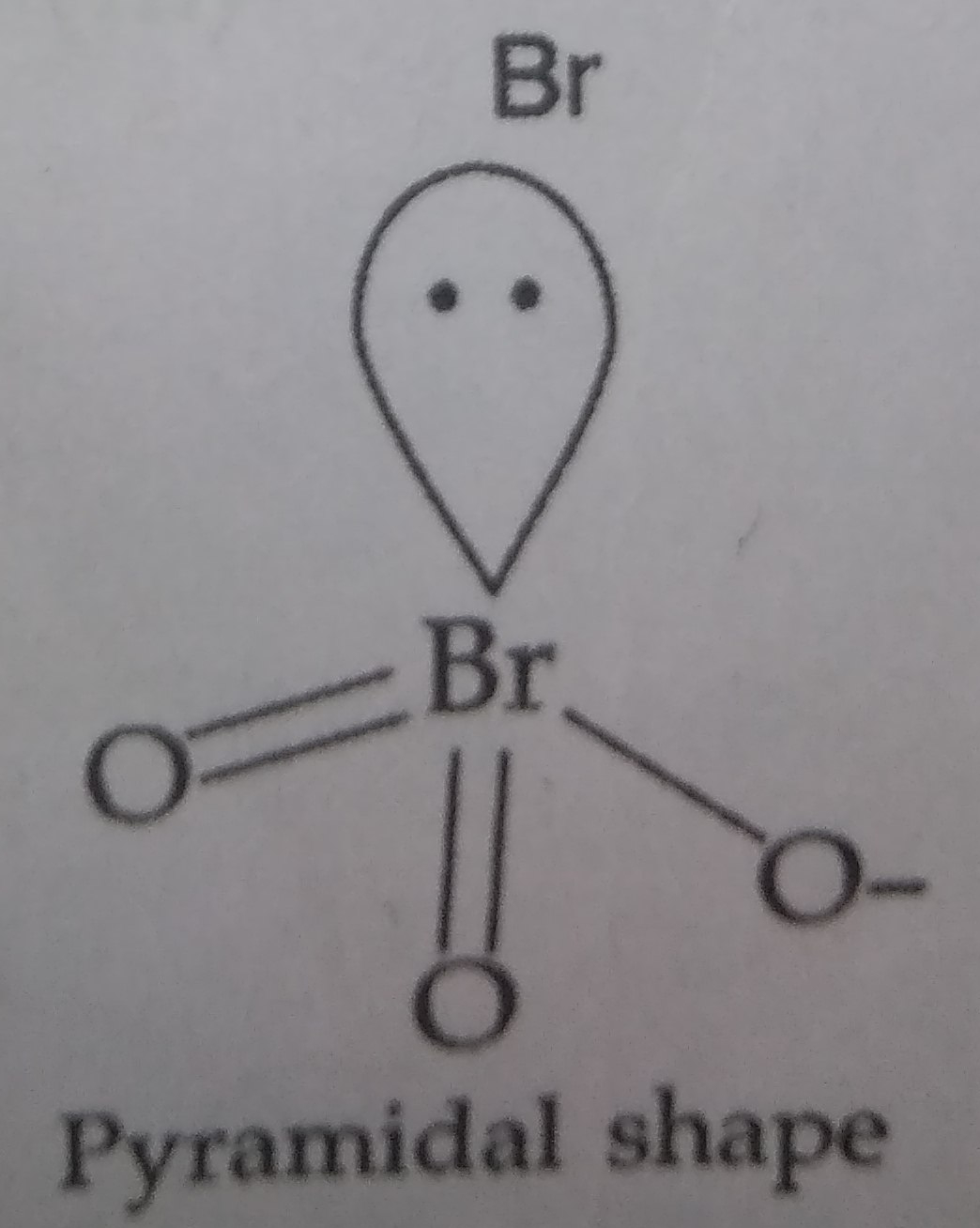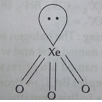(a) Write the formula and describe the structure of a noble gas species which is isostructural with

(i) $IB{r}_{2}^{-}$                                                           (ii) $Br{O}_{3}^{-}$

(b) Assign reasons for the following :

(i) $S{F}_{6}$ is kinetically inert.

(ii) $N{F}_{3}$ is an exothermic compound whereas $NC{l}_{3}$ is not.

(iii) HCl is a stronger acid than HF though flourine is more electronegative than chlorine.

Concept Videos :-

#7 | 17th Group

Concept Questions :-

group 17 ,preparation and properties

(a) (i) $IB{r}_{2}^{-}$ : It has 2 bond pairs and 3 lone pairs. Therefore according to VSEPR theory it should have Linear structure. $IB{r}_{2}^{-}$ has (7+2$×$7+1) i.e. 22 valence electrons. No noble gas has 22 electrons

$\therefore$ The isoelectronic species for(ii) $Br{O}_{3}^{-}$ : It has 3 bond pairs and 1 lone pair of electrons. Therefore according to VSEPR theory it has pyramidal structure.

It has 26 valence electrons i.e. (7+3$×$6+1=26).A noble gas having 26 valence electrons is $Xe{O}_{3}$ (8+3$×$6=26). Thus $Xe{O}_{3}$ also has pyramidal structure.(b) (i) Because $S{F}_{6}$ is showing steric hindrance due to 6 (six) fluorine atoms which make it unable to react further with any other atom.

(ii) Due to smaller size of F as compared to Cl, the N-F bond is much stronger than N-Cl bond while bond dissociation energy of ${F}_{2}$ is much lower than that of $C{l}_{2}$. Therefore, energy released during the formation of $N{F}_{3}$ molecules is more than the energy needed to break ${N}_{2}$ and ${F}_{2}$ molecules into individual atoms. In other words, formation of $N{F}_{3}$ is an exothermic reaction.

The energy released during the formation of $NC{l}_{3}$ molecule is less than the energy needed to break ${N}_{2}$ and $C{l}_{2}$ molecule into individual atoms. Thus formation of $NC{l}_{3}$ is an endothermic  reaction.

(iii) Because HCl has large size and less bond strength than HF which makes easier liberation of ${H}^{+}$.

Difficulty Level: